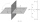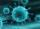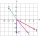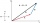# Points collinear

Show that the point A(-1,3), B(3,2), C(11,0) are col-linear.

Result

x =  1

#### Solution:Leave us a comment of example and its solution (i.e. if it is still somewhat unclear...):

Showing 0 comments:Be the first to comment!#### To solve this example are needed these knowledge from mathematics:

For Basic calculations in analytic geometry is helpful line slope calculator. From coordinates of two points in the plane it calculate slope, normal and parametric line equation(s), slope, directional angle, direction vector, the length of segment, intersections the coordinate axes etc. Two vectors given by its magnitudes and by included angle can be added by our vector sum calculator.

## Next similar examples:

1. Vector - basic operationsThere are given points A [-9; -2] B [2; 16] C [16; -2] and D [12; 18] a. Determine the coordinates of the vectors u=AB v=CD s=DB b. Calculate the sum of the vectors u + v c. Calculate difference of vectors u-v d. Determine the coordinates of the vector w.
2. Symmetry by planeDetermine the coordinates of a image of point A (3, -4, -6) at a symmetry that is determined by the plane x-y-4z-13 = 0
3. LineLine p passing through A[-10, 6] and has direction vector v=(3, 2). Is point B[7, 30] on the line p?
4. VirusWe have a virus that lives one hour. Every half hour produce two child viruses. What will be the living population of the virus after 3.5 hours?
5. LineIt is true that the lines that do not intersect are parallel?
6. Add vectorGiven that P = (5, 8) and Q = (6, 9), find the component form and magnitude of vector PQ.
7. Scalar productCalculate the scalar product of two vectors: (2.5) (-1, -4)
8. Scalar dot productCalculate u.v if |u| = 5, |v| = 2 and when angle between the vectors u, v is: a) 60° b) 45° c) 120°
9. VectorDetermine coordinates of the vector u=CD if C[19;-7], D[-16,-5].
10. AS sequenceIn an arithmetic sequence is given the difference d = -3 and a71 = 455. a) Determine the value of a62 b) Determine the sum of 71 members.
11. CalculationHow much is sum of square root of six and the square root of 225?
12. Parametric equationFind the parametric equation of a line with y-intercept (0,-4) and a slope of -2.
13. Theorem proveWe want to prove the sentense: If the natural number n is divisible by six, then n is divisible by three. From what assumption we started?
14. Coordinates of vectorDetermine the coordinate of a vector u=CD if C(19;-7) and D(-16;-5)
15. Quadratic equationFind the roots of the quadratic equation: 3x2-4x + (-4) = 0.
16. EquationEquation ? has one root x1 = 8. Determine the coefficient b and the second root x2.
17. Fish tankA fish tank at a pet store has 8 zebra fish. In how many different ways can George choose 2 zebra fish to buy?